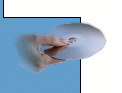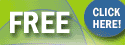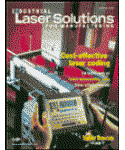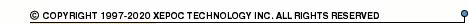More than478 791 registered clientsJWC00603S1V5 series datasheets. Manufacturer: IPSI.

 JWC00603S1V5 Non-isolated DC/DC converter. Input voltage 3.3V. Nominal output voltage 1.5V. Output current: 0.0A (min). 6.0A (max). in 4-pin - package. Operational temperature range from 0°C to 80°C. Datasheet*) JWC00603S1V8 Non-isolated DC/DC converter. Input voltage 3.3V. Nominal output voltage 1.8V. Output current: 0.0A (min). 6.0A (max). in 4-pin - package. Operational temperature range from 0°C to 80°C. Datasheet*) JWC00603S2V0 Non-isolated DC/DC converter. Input voltage 3.3V. Nominal output voltage 2.0V. Output current: 0.0A (min). 6.0A (max). in 4-pin - package. Operational temperature range from 0°C to 80°C. Datasheet*) JWC00603S2V5 Non-isolated DC/DC converter. Input voltage 3.3V. Nominal output voltage 2.5V. Output current: 0.0A (min). 6.0A (max). in 4-pin - package. Operational temperature range from 0°C to 80°C. Datasheet*) JWC00605S1V5 Non-isolated DC/DC converter. Input voltage 5.0V. Nominal output voltage 1.5V. Output current: 0.0A (min). 6.0A (max). in 4-pin - package. Operational temperature range from 0°C to 80°C. Datasheet*) JWC00605S1V8 Non-isolated DC/DC converter. Input voltage 5.0V. Nominal output voltage 1.8V. Output current: 0.0A (min). 6.0A (max). in 4-pin - package. Operational temperature range from 0°C to 80°C. Datasheet*) JWC00605S2V0 Non-isolated DC/DC converter. Input voltage 5.0V. Nominal output voltage 2.0V. Output current: 0.0A (min). 6.0A (max). in 4-pin - package. Operational temperature range from 0°C to 80°C. Datasheet*) JWC00605S2V5 Non-isolated DC/DC converter. Input voltage 5.0V. Nominal output voltage 2.5V. Output current: 0.0A (min). 6.0A (max). in 4-pin - package. Operational temperature range from 0°C to 80°C. Datasheet*) JWC00605S3V3 Non-isolated DC/DC converter. Input voltage 5.0V. Nominal output voltage 3.3V. Output current: 0.0A (min). 6.0A (max). in 4-pin - package. Operational temperature range from 0°C to 80°C. Datasheet*) JWC00603S1V5-L Non-isolated DC/DC converter. Input voltage 3.3V. Nominal output voltage 1.5V. Output current: 0.0A (min). 6.0A (max). in 4-pin - package. Operational temperature range from 0°C to 80°C. Datasheet*) JWC00603S1V8-L Non-isolated DC/DC converter. Input voltage 3.3V. Nominal output voltage 1.8V. Output current: 0.0A (min). 6.0A (max). in 4-pin - package. Operational temperature range from 0°C to 80°C. Datasheet*) JWC00603S2V0-L Non-isolated DC/DC converter. Input voltage 3.3V. Nominal output voltage 2.0V. Output current: 0.0A (min). 6.0A (max). in 4-pin - package. Operational temperature range from 0°C to 80°C. Datasheet*) JWC00603S2V5-L Non-isolated DC/DC converter. Input voltage 3.3V. Nominal output voltage 2.5V. Output current: 0.0A (min). 6.0A (max). in 4-pin - package. Operational temperature range from 0°C to 80°C. Datasheet*) JWC00605S1V5-L Non-isolated DC/DC converter. Input voltage 5.0V. Nominal output voltage 1.5V. Output current: 0.0A (min). 6.0A (max). in 4-pin - package. Operational temperature range from 0°C to 80°C. Datasheet*) JWC00605S1V8-L Non-isolated DC/DC converter. Input voltage 5.0V. Nominal output voltage 1.8V. Output current: 0.0A (min). 6.0A (max). in 4-pin - package. Operational temperature range from 0°C to 80°C. Datasheet*) JWC00605S2V0-L Non-isolated DC/DC converter. Input voltage 5.0V. Nominal output voltage 2.0V. Output current: 0.0A (min). 6.0A (max). in 4-pin - package. Operational temperature range from 0°C to 80°C. Datasheet*) JWC00605S2V5-L Non-isolated DC/DC converter. Input voltage 5.0V. Nominal output voltage 2.5V. Output current: 0.0A (min). 6.0A (max). in 4-pin - package. Operational temperature range from 0°C to 80°C. Datasheet*) JWC00605S3V3-L Non-isolated DC/DC converter. Input voltage 5.0V. Nominal output voltage 3.3V. Output current: 0.0A (min). 6.0A (max). in 4-pin - package. Operational temperature range from 0°C to 80°C. Datasheet*)Industrial Laser Solutions for Manufacturing Magazine.Subscribe now for FREE !